# The Salty Economist

Things I Should Have Learned in High School

## F# Testing with xUnit

I came across a cool tool yesterday for testing F# programs called xUnit and I thought I should give it a try, so here goes.

The first step:  Get it and install it.

Don't worry about any documentation there is none -- you've just got to wing it.  I downloaded the release and unzipped it: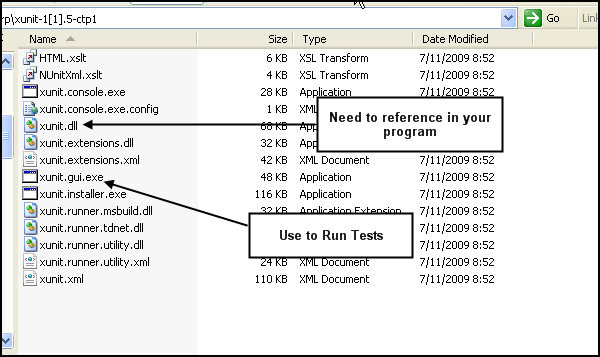You don't need to do any more that that.

The next thing I did was add a reference to the xunit.dll file: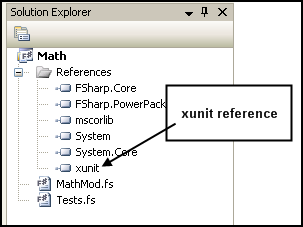Now for our first test.  In my last post, I showed how to code a mortgage calculator.  I now want to write a test to prove that it works.

The first thing I did was I took my present value function (along with my other math functions) and stowed them away in a module called MathMod.

Here is the relevant section of MathMod:

-------------------------------------------------------------

module MathMod

#light
open System
open System.Collections.Generic
open System.IO

let pv (i : float) (n : float) =
1.0 / Math.Pow( (1.0 + i ), n)

let npv x i n =
x * (pv i n)

let rec valList aList (ir : float) (n : float) =
match aList with
| [] -> []
| h::t -> npv h ir n :: valList t ir (n+1.0)

let presentValue vals (ir : float) =
List.fold(+)0.0 <| valList vals ir 0.0

----------------------------------------------------------------------

I next added a new file to my project called "Tests.fs".  I figured I would put all my tests in this file so I could then run them all at once.

Now, the concept behind xUnit is that you

(1) create an .exe or .dll that contains some tests;

(2) compile the .exe or .dll; and then

(3) run an axillary program "Test Runner.exe" that looks in your .exe or .dll for methods that are tagged as tests.  The Test Runner  then runs the tests and notifies you if they have succeeded or failed.  Of course, the Test Runner program is not called that, but rather it is officially named xunit.gui.exe (see above).

So, let's try something simple -- this is what my Test.fs file looks like:

----------------------------------------------------------------------

#light

open System
open System.Collections.Generic
open System.IO
open Xunit
open MathMod

[<Fact>]
let PV_1000() =
let vals = [-1000.0; 100.0; 100.0; 100.0; 100.0; 100.0; 100.0; 100.0; 100.0; 100.0; 1100.0]
let pv0 = presentValue vals 0.1
Assert.InRange(pv0,(0.0 - 0.0001),(0.0 + 0.0001))

----------------------------------------------------------------------

Notice, I open Xunit (note the capitalization).  I don't know why xUnit is called Xunit ???

Anyway, a test is structured with a tag  [<Fact>].

You then define a method -- I call it PV_1000() in this example.

Caution:  You must append the open parentheses at the end of the function, otherwise the Test Runner won't identify it as a test.  The program will still compile, it's just that the Test Runner won't find it.  It only took me two friggin' hours to figure this out.

In my test I have a series of numbers whose present value I know should be zero (0.0).  The statement:

Assert.InRange(pv0,(0.0 - 0.0001),(0.0 + 0.0001))

Asserts that the result of the present value calculation (pv0) is in the range of 0 +/- 0.0001.  (I can't use equality here because I am using floating point numbers.)  The Test Runner application will find any method tagged as tag  [<Fact>] and run it.  The Assert statement will be executed and will succeed or fail.

So, let's try it.  I first compile the program in F#.  I then run the Test Runner (xunit.gui.exe).  I then open my assembly: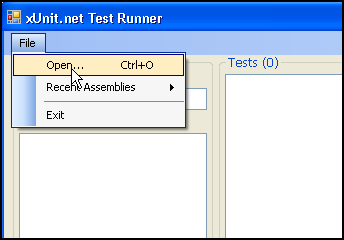When I open my assembly, the Test Runner shows the tests it has identified.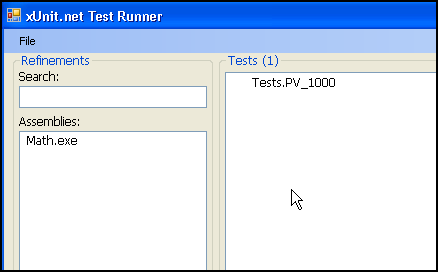To run, the test(s), I just click the run button.

The Test Runner then runs the test and indicates if it has passed or failed: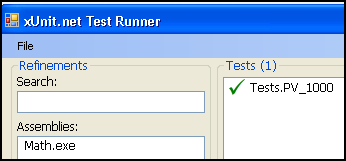Here it has passed.

Now here is a test I know will fail:

[<Fact>]
let PV_1000_Fail() =
let vals = [-1000.0; 100.0; 100.0; 100.0; 100.0; 100.0; 100.0; 100.0; 100.0; 100.0; 1100.0]
let pv0 = presentValue vals 0.12
Assert.InRange(pv0,(0.0-0.0001),(0.0+0.0001))

I have changed the discount rate to 12% which should make the present value less than zero.  I recompile the program and run the Test Runner.

Here's what a failure looks like: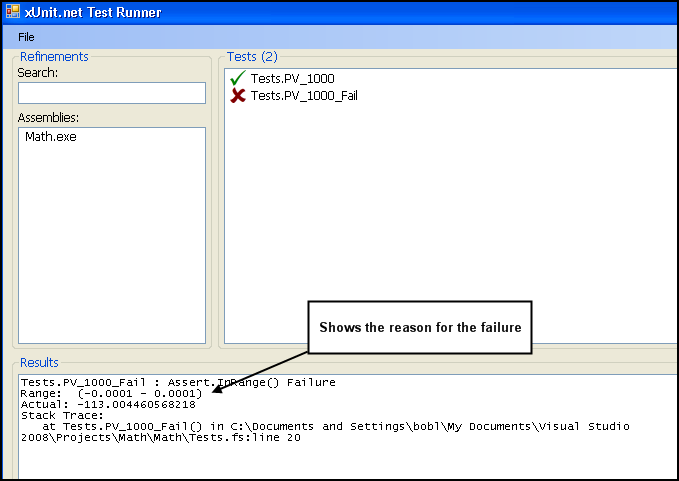The results screen show the failure and the reason it failed.  Note that the present value is now -113.00 -- this value fails the test.

Cool Huh?

Now, the test I really want to write is a test of my mortgage calculator:

-------------------------------------------------------------

[<Fact>]
let mortgageCalc() =

let mortgage = 200000.0
let n = 360
let i = 0.06

let ones = List.init n (fun one -> 1.0)

let pv0 = presentValue ones (i/12.0)

let levelPayment = mortgage / pv0

//
//Now Test
//
let payments = List.init n (fun one -> levelPayment)

let pv2 = presentValue payments (i/12.0)
Assert.InRange(pv2,(mortgage-0.0001),(mortgage+0.0001))

-------------------------------------------------------------

In this test, I calculate my level payment for a \$200,000 mortgage over 360 months at 6.00% interest.  I then calculate create a stream of payments equal to my level payment.  I then discount that stream back to the present.  The result is pv2.  I then Assert that the result pv2 is equal (within a tolerance) of my original mortgage.

I compile and run.

We Pass!

Print | posted on Sunday, July 12, 2009 11:28 AM |